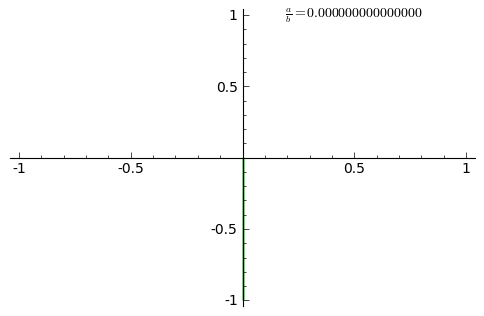# Lissajous Curves (Bowditch Curve)

Lissajous curves are created by two simple harmonic motions — combinations of sine functions and/or cosine functions.

The curves are named after Jules-Antoine Lissajous, who studied them in 1857. They are sometimes called Bowditch curves after Nathaniel Bowditch, who discovered them (independently) in 1815 while studying a compound’s pendulum movement .

Lissajous curves are useful for studying the mechanical problem of small oscillations with two degrees of freedom: the system of differential equations x′′ = –y(t), y′′(t) = –n2x(t), where n > 0,

## Lissajous Curve Equations

The parametric equations are combinations of sines and cosines:

• sin(nt + a), cos(kt + b) or
• sin(nt + a), sin(kt + b) or
• cos(nt + a), cos(kt + b) or

It’s common for the curves to be described by equations where a + b = 0. For example,

• x = cos(nθ), sin(k&theta)

You can play around with various values for n and k with this Desmos calculator page (which I used to create the top image).

## Properties of the Lissajous Curve

The constants n and k determine the curve’s size.

One oscillation determines the x-coordinate and the other the y-coordinate.

When the ratio of the two oscillations’ frequencies is rational number (i.e., a/b) the curve is a closed curve. Different shapes are created with different ratios . When the frequencies are equal, the curve is a line segment (if the difference in phases is a multiple of π) or an ellipse.Animation of Lissajous curves with increasing a/b ratio from 0.0 to 1.0 in steps of 0.01 .

There are two types of Lissajous curves :

Lissajous curves are Chebyshev polynomials , under certain conditions:

• n = 1,
• k = a natural number, and
•.

Despite these straightforward properties, predicting the shape of the curve is challenging and may require some experimentation to achieve a certain shape .

## References

 L.R. Ingersoll Physics Museum. Lissajous Curve. Retrieved February 28, 2022 from: https://www.physics.wisc.edu/ingersollmuseum/exhibits/waves/lissajous/
 MacTutor. Lissajous Curves. Retrieved February 28, 2022 from: https://mathshistory.st-andrews.ac.uk/Curves/Lissajous/
 Krishnavedala, ,CC BY-SA 3.0 via Wikimedia Commons
 Project: Lissajous Figures. Retrieved February 28, 2022 from: http://mathserver.neu.edu/~bridger/U170/Lissajous/Lissajous.htm
 Merino, J. (2003). Lissajous Figures and Chebyshev Polynomials. The College Mathematics Journal.
 Seggern, D. (1994). PHB practical handbook of curve design and generation. CRC Press.

CITE THIS AS:
Stephanie Glen. "Lissajous Curves (Bowditch Curve)" From StatisticsHowTo.com: Elementary Statistics for the rest of us! https://www.statisticshowto.com/lissajous-curves/